Technical Article

# Understanding DC Circuit Capacitor Behavior

November 09, 2023 by Amna Ahmad

## This article discusses the fundamental concepts governing capacitors' behavior within DC circuits. Learn about the time constant and energy storage in DC circuit capacitors and the dangers associated with charged capacitors.

Capacitors are insulators, so the current measured in any circuit containing capacitors is the movement of the free electrons from the positive side of a capacitor to the negative side of that capacitor or another capacitor. The current does not flow through the capacitor, as current does not flow through insulators. When the capacitor voltage equals the battery voltage, there is no potential difference, the current stops flowing, and the capacitor is fully charged. If the voltage increases, further migration of electrons from the positive to negative plate results in a greater charge and a higher voltage across the capacitor.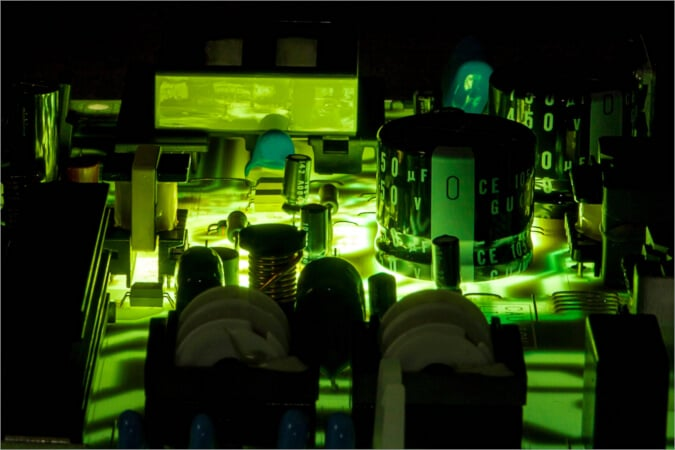##### Image used courtesy of Adobe Stock

Figure 1 illustrates a capacitor connected to a battery. When first connected, the capacitor would have no charge, meaning the number of free electrons on either side of the capacitor would be approximately equal. The capacitor would begin to charge, with the positive plate of the battery attracting some of the free electrons from the capacitor, causing the connected capacitor plate to become positively charged.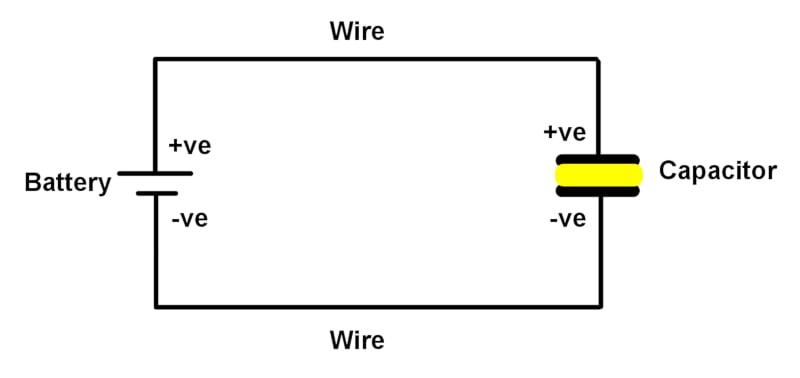##### Figure 1. A capacitor is connected to a battery. Image used courtesy of Amna Ahmad

The other plate of the capacitor, connected to the battery's negative, would receive the free electrons displaced from the other side of the capacitor, becoming negatively charged.

### Time Constant

The rate at which a capacitor is charged depends on the capacitance and the circuit resistance.

The formula to calculate the charge is:

$Q=CV=It$

Since t = CV/I and R = V/I

Therefore

$\tau=RC$

where

$$\tau$$ = charge time for the capacitor in seconds (one ‘time constant’)

R = resistance in ohms

### What Kind of Time is τ?

The time constant is usually denoted by the Greek letter tau or τ, which is the capacitor's time to charge up to 63 percent of the applied voltage.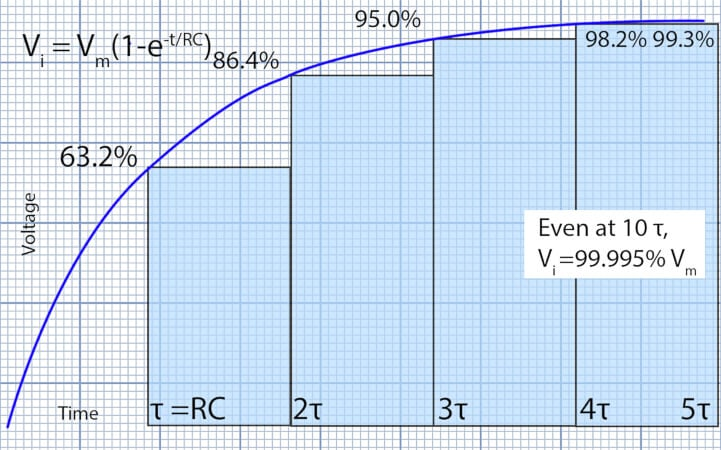##### Figure 2. Capacitor charging voltage. Image used courtesy of Amna Ahmad

Example 1

A circuit consists of a 100 kΩ resistor in series with a 500 µF capacitor. How long would it take for the voltage across the capacitor to reach 63% of the value of the supply?

$\tau=RC=100E+3\times500E-6=50s$

Therefore, to increase the charging time, either the capacitance or the resistance must increase. Likewise, decreasing either value decreases the time constant.

Notice the formula does not include voltage or current. The supply voltage does not affect the charging time for any given capacitor. Doubling the supply voltage doubles the charging current, but the electric charge pushed into the capacitor is also doubled, so the charging time remains the same. Plotting the voltage values against time for any capacitor charging from a constant voltage results in an exponential curve increasing toward the applied voltage.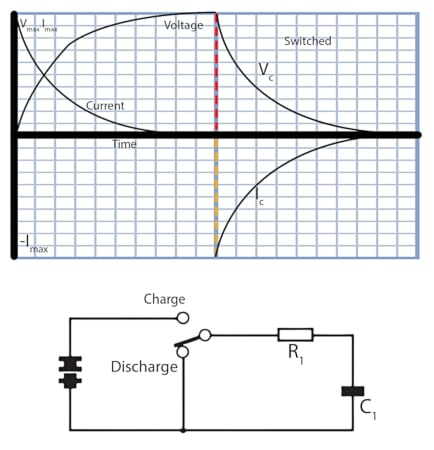##### Figure 3. Capacitor charge/discharge. Image used courtesy of Amna Ahmad

Discharging a capacitor into a fixed resistance creates another exponential curve, this time reducing toward zero.

The discharge current is a negative value because of the reversal of current flow. The charge flows out of the capacitor.

The curves show the current is at a maximum when the voltage changes rapidly (i.e., at the start of charging and discharging).

The current is zero when the voltage is steady, but technically, the voltage never quite reaches a maximum, as exponential curves continue to rise for infinity (the voltage never reaches a steady value).

When the circuit resistance value is very small, extremely high current values can result, and the charging time may be reduced to millionths of a second. By Q = VC = IT, when the discharge time is small, high currents must flow. This high discharge current can be dangerous.

Because no dielectric is a perfect insulator, a charged capacitor will slowly lose its charge as current leaks from one plate to another. However, a really good capacitor may hold its charge for a very long time.

Therefore, to reduce electric shock risk, many high-voltage, high-power circuits have a high-value bleed resistor connected across the capacitor to reduce the charge to a safe limit within perhaps ten seconds (see Figure 4).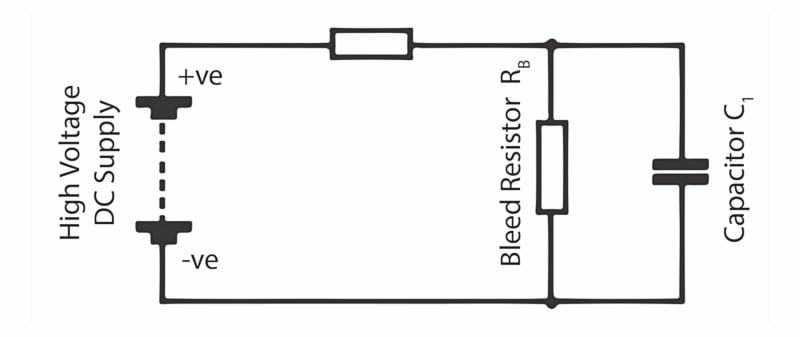##### Figure 4. Capacitor charging circuit. Image used courtesy of Amna Ahmad

Knowing that the time a capacitor takes to charge or discharge to a set voltage can be calculated from resistance and capacitance, a circuit can be designed to operate at that value, perhaps to turn a light on or off or control how long a motor runs or takes to start.

### Energy Stored in a Capacitor

When a capacitor is charged, a static electric field exists between the plates. This results from the electrons being pumped from the positive to the negative plate and the attraction between them and their counterpart positive ions. The actual value of stored energy depends on the capacity and voltage of the capacitor.

Unlike an inductor, which must have a dynamic flow of electrons (a current) to maintain its charge, a capacitor needs only a stored (static) charge of electrons. The attraction between the electrons and positive ions keeps the electrons in place, and the capacitor remains charged until leakage allows the charge to escape.

The actual energy value stored in the field depends on the applied voltage and capacitance. Determine the energy stored in a capacitor using the formula:

$W=\frac{1}{2}CV^{2}$

Example 2

A 1 µF capacitor is charged from a 300 V DC supply. Find the energy stored in the capacitor.

$W=\frac{1}{2}CV^{2}=\frac{1}{2}\times1E-6\times300^{2}=0.045J(45mJ)$

### Charged Capacitor Dangers

The value of energy stored in the capacitor in Example 2 is certainly low. However, because the potential difference across the terminals is 300 V, an operator can get an unpleasant, if not dangerous, electric shock.

Capacitors can store the charge for a long time after the supply has been disconnected. A capacitor used on three-phase line voltages can have a charge exceeding 500 V. Electric circuits such as modern switch-mode welders can have large capacitors, charged well above the supply voltage, still alive even after the plug has been removed from the socket. Electrical engineers should always maintain care when dealing with capacitors.

Depending on actual circumstances, a high discharge current can flow from a charged capacitor. To illustrate this, consider Example 3.

Example 3

A resistor of 0.4 Ω is connected across the terminals of a 1 μF capacitor while it is charged to 300 VDC. What current will flow through the capacitor and resistor?

From Ohm's law:

$I=\frac{V}{R}=\frac{300}{0.4}=750A$

This current lasts briefly before deteriorating to a much lower value. Still, it can cause circuit damage if the circuit or the capacitor is not built to withstand such current surges.

A circuit of this type is the basis of photographic flash guns, which generate a high current for a few milliseconds to generate the bright electric arc you see as a camera flash. A capacitor is charged up to 200–500 V and discharged into a xenon gas–filled tube.

Before handling capacitors or working on circuits where capacitors are used, it is a sensible precaution to ensure they have been discharged. Small capacitors can be discharged directly with a short circuit. Still, where there is a safety issue, larger values might need a discharge (bleed) resistor to control the current value during discharge.

Some circuits have high-value ‘bleed’ resistors permanently connected across a capacitor to ensure a controlled discharge. This applies particularly in higher voltage circuits.

### DC Circuit Capacitor Takeaways

In DC circuits, capacitors play a crucial role. The time constant, determined by the capacitance and resistance in the circuit, governs the charging and discharging behavior of the capacitor. Understanding the time constant helps in analyzing the transient response and determining the rate at which the capacitor reaches its final voltage or discharges to zero.

Capacitors are also known for their ability to store electrical energy, which can be released when needed. However, it is important to be cautious of the dangers associated with charged capacitors, as they can retain a significant amount of electrical energy.